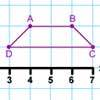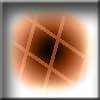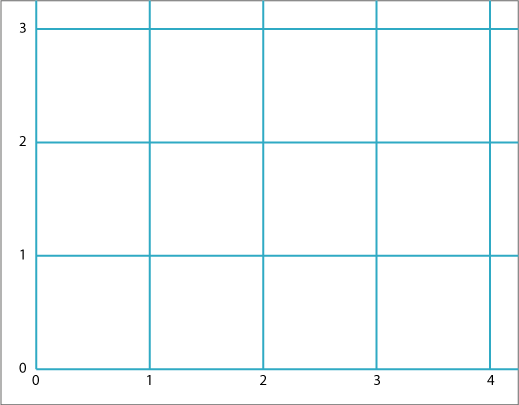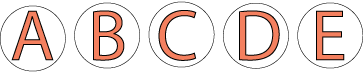#### You may also like### Clock Hands

This investigation explores using different shapes as the hands of the clock. What things occur as the the hands move.### Transformation Tease

What are the coordinates of this shape after it has been transformed in the ways described? Compare these with the original coordinates. What do you notice about the numbers?### Penta Play

A shape and space game for 2, 3 or 4 players. Be the last person to be able to place a pentomino piece on the playing board.

# Coordinate Challenge

## Coordinate Challenge

Here is a grid:Can you position these ten letters in their correct places according to the eight clues below?Clues:

The letters at $(1, 1),$ $(1, 2)$ and $(1, 3)$ are all symmetrical about a vertical line.

The letter at $(4, 2)$ is not symmetrical in any way.

The letters at $(1, 1),$ $(2, 1)$ and $(3, 1)$ are symmetrical about a horizontal line.

The letters at $(0, 2),$ $(2, 0)$ have rotational symmetry.

The letter at $(3, 1)$ consists of just straight lines.

The letters at $(3, 3)$ and $(2, 0)$ consist of just curved lines.

The letters at $(3, 3),$ $(3, 2)$ and $(3, 1)$ are consecutive in the alphabet.

The letters at $(0, 2)$ and $(1, 2)$ are at the two ends of the alphabet.

### Why do this problem?

There are two main parts to this problem. Firstly, learners need to identify the symmetries of various capital letters. Secondly, they need to practise reading coordinates. The problem should be approached systematically because some of the clues are not straightforward.

### Possible approach

It would be good to have the grid displayed on an interactive whiteboard or data projector so that you can annotate the screen throughout the lesson. Start by drawing some of the letters on the grid and asking the children a few questions about where they are placed which will remind them about symmetry and coordinates. For example, you could ask "Where is the letter that has two lines of symmetry?"; "What do all the letters at ... have in common?".

After this learners could work in pairs on the problem so that they are able to talk through their ideas with a partner. They could use this sheet which has letters that can be cut out and moved around. Alternatively, the problem could be tackled on squared paper and the letters drawn on in pencil so that they can be altered easily.

If pupils do not have much experience of dealing with clues and prioritising their order as this problem requires, it might be appropriate to read each clue in turn as a whole group before working on the task in pairs. This way, you can reassure the learners that it doesn't matter if you cannot immediately use a clue to place a letter definitively. You can also demonstrate that having the letters to move around, or writing them on the grid in pencil, means that the solution can be refined as more information is revealed.

### Key questions

What kind of symmetry does this letter have?
Where could this letter go on the grid? How do you know?
Which letters fit this clue?

### Possible extension

Learners could explore and list the symmetries of all the other letters of the alphabet.

### Possible support

Children could start by identifying the symmetries of the letters, listing whether they have vertical or horizontal line symmetry, rotational symmetry or no symmetry at all. They can then refer to their list as they try to place letters on the grid.